• LR1状态图构造与LR1与LALR分析构造的Flash程序与代码（包含.fla,.as,.exe文件） ●LR1状态图构造 →输入文法，可以构造出LR1状态图 →可以对状态图用张力-斥力模型自动布局 →点击状态编号以高亮显示该状态...
• LR(0)完整的语法分析方法(拓广文法、识别活前缀状态机、LR0、识别字符串)内有word详细说明
• 一个LR分析器的工作过程可看成是栈里的状态序列，已规约串和输入串所构成的三元式的变化过程。分析开始时的初始三元式为 (s0, #, a1a2……an#) 其中，s0为分析器的初态；＃为句子的左括号；a1a2……an为输入串；其...
• 1． 根据给定文法和LR分析，构造LR分析器，并要求输出LR工作过程。 2． 根据LR分析器分析过程算法，程序能够准确输出LR分析器工作过程。通过action()和goto_char()两个函数即可实现算法的功能。另外，还需开辟...
• 本节，我们探讨如何通过LALR(1)有限状态机构建能用于自底向上语法分析的跳转
阅读博客的朋友可以到我的网易云课堂中，通过视频的方式查看代码的调试和执行过程：

http://study.163.com/course/courseMain.htm?courseId=1002830012

如果大家运行上一节的代码，可以得到压缩后的LR有限状态机，以及节点间的跳转关系：

***begin to print a map row***

from state:
State Number: 0
STMT -> .EXPR look ahead set: { EOI }
on symbol: TERM
to state:
State Number: 1
EXPR -> TERM .look ahead set: { EOI }
TERM -> TERM .TIMES FACTOR look ahead set: { EOI }
TERM -> TERM .TIMES FACTOR look ahead set: { TIMES }
EXPR -> TERM .look ahead set: { PLUS }
TERM -> TERM .TIMES FACTOR look ahead set: { PLUS }
EXPR -> TERM .look ahead set: { RIGHT_PARENT }
TERM -> TERM .TIMES FACTOR look ahead set: { RIGHT_PARENT }
on symbol: EXPR
to state:
State Number: 2
STMT -> EXPR .look ahead set: { EOI }
EXPR -> EXPR .PLUS TERM look ahead set: { EOI }
EXPR -> EXPR .PLUS TERM look ahead set: { PLUS }
on symbol: NUM_OR_ID
to state:
State Number: 3
FACTOR -> NUM_OR_ID .look ahead set: { EOI }
FACTOR -> NUM_OR_ID .look ahead set: { TIMES }
FACTOR -> NUM_OR_ID .look ahead set: { PLUS }
FACTOR -> NUM_OR_ID .look ahead set: { RIGHT_PARENT }
on symbol: LEFT_PARENT
to state:
State Number: 4
FACTOR -> LEFT_PARENT .EXPR RIGHT_PARENT look ahead set: { EOI }
FACTOR -> LEFT_PARENT .EXPR RIGHT_PARENT look ahead set: { TIMES }
FACTOR -> LEFT_PARENT .EXPR RIGHT_PARENT look ahead set: { PLUS }
FACTOR -> LEFT_PARENT .EXPR RIGHT_PARENT look ahead set: { RIGHT_PARENT }
on symbol: FACTOR
to state:
State Number: 5
TERM -> FACTOR .look ahead set: { EOI }
TERM -> FACTOR .look ahead set: { TIMES }
TERM -> FACTOR .look ahead set: { PLUS }
TERM -> FACTOR .look ahead set: { RIGHT_PARENT } ***end a map row***

上面是程序输出的一部分结果，它表明了从节点0如何跳转到其他节点，例如，从上面的节点可以看出，当处于节点0的时候，在输入为t(term) 时，跳转到节点1，以此类推。

在上面的信息输出中，只表明了节点间的跳转关系，有另一层信息并没有显示出来，那就是当处于某个节点时，是否要采取reduce操作。

我们看节点3，点符号处于节点表达式的末尾，当状态机处于该节点，并且当输入属于EOI，TIMES，PLUS，RIGHT_PARENT，
其中之一时，我们应该采取reduce操作，在我们程序的输出信息中，reduce操作的相关信息并没有显示，因此，要构造一个完整的跳转表，我们还需要构建每个节点的reduce信息。

reduce信息的构建不难，只要遍历每个节点，查看节点包含的表达式，如果符号”.”位于表达式的末尾，那么该节点即可根据该表达式以及表达式对应的look ahead 集合，打印相关的reduce信息。在GrammarState中，我添加了一个reduce函数，它的作用是构建对应节点的reduce信息：


private void  reduce(HashMap<Integer, Integer> map, ArrayList<Production> productions) {
for (int i = 0; i < productions.size(); i++) {
if (productions.get(i).canBeReduce()) {
ArrayList<Integer> lookAhead = productions.get(i).getLookAheadSet();
for (int j = 0; j < lookAhead.size(); j++) {
map.put(lookAhead.get(j), (productions.get(i).getProductionNum()));
}
}
}
}

代码的逻辑，大家可观看稍后的代码解读。

有了节点的跳转信息，和reduce信息，我们就可以构建完整的状态跳转表了。

跳转表的构建在GrammarStateManager中，在该类中有一个成员变量：

HashMap<Integer, Map<Integer, Integer>> lrStateTable.

这个变量是一个间套的HashMap, 红色的Integer表示当前节点的编号，蓝色的Integer表示对应的输入符号所对应的数值，第三个紫色的Integer代表两个含义，如果它是大于0的正数，那表明，从红色Integer表示的节点跳转到紫色的Integer所表示的节点，如果它是0或负数，表明当处于红色Integer的节点时，要做一次reduce操作，举个例子：
Integer: 3
Integer: 0 (EOI)
Integer: -6

上面的数据表明，当处于节点3，输入是EOI时，根据表达式6(f->NUM)做一个reduce操作.于是当解析器在解析时，将当前所在的状态节点号，当前输入符号的数值在上面的跳转表中查找，如果得到的第三更Integer是正数，那么解析器就跳转到指定节点，如果得到的是负数，那么解析器就根据相应数值做一次reduce操作。
结合跳转关系和reduce信息后，我们构造的状态机跳转表如下：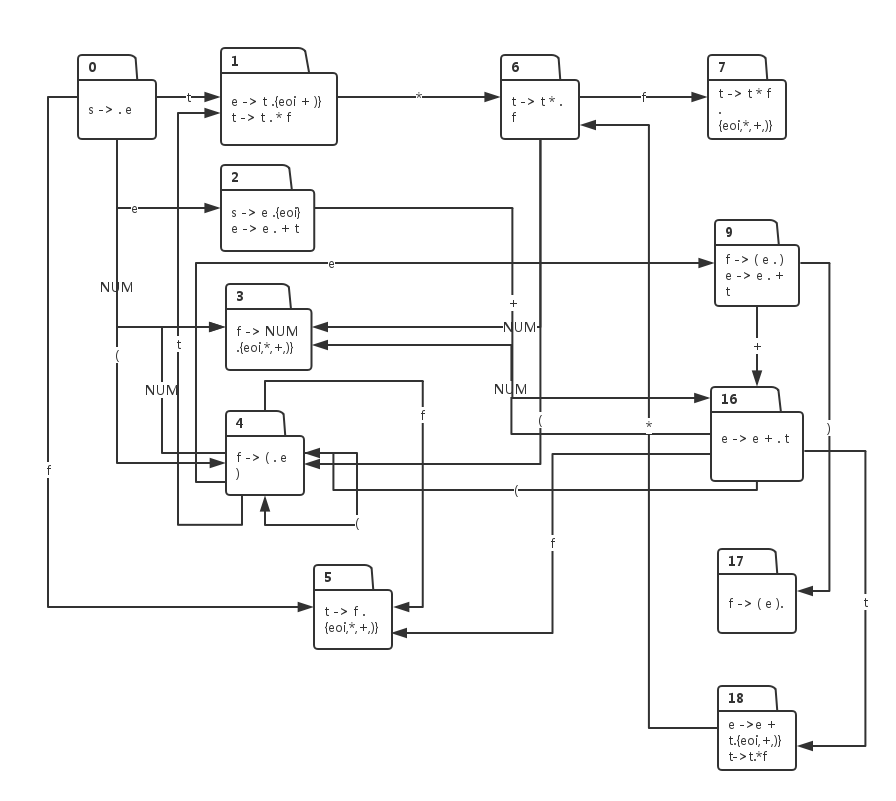大家或许发现，上面的状态机图跟我们最早构造的状态机图其实是一模一样的，只不过一些节点的编号变了，同时能做reduce操作的节点中，添加了对应的look ahead 集合。

接下来我们看看相关代码的实现:

阅读博客的朋友可以到我的网易云课堂中，通过视频的方式查看代码的调试和执行过程：

http://study.163.com/course/courseMain.htm?courseId=1002830012
展开全文java 编译器
• ## LR(0)分析表的构造

千次阅读 2020-11-29 00:21:06
LR分析的构造活前缀构造识别活前缀的DFA文法的拓广将文法G(S)G(S)G(S)拓广为G′(S′)G'(S')G′...状态转换函数示例LR(0)项目集规范族的构造算法示例构造LR(0)分析的算法LR(0)分析的构造构造LR(0)分析的算法示例...
LR分析表的构造活前缀构造识别活前缀的DFA文法的拓广将文法$G(S)$拓广为$G'(S')$LR(0)项目构造识别文法所有活前缀的DFA通过计算项目集规范族构造识别活前缀的DFA有效项目有效项目的性质LR(0)项目集规范族的构造项目集的闭包CLOSURE状态转换函数示例LR(0)项目集规范族的构造算法示例构造LR(0)分析表的算法LR(0)分析表的构造构造LR(0)分析表的算法示例
活前缀

活前缀：是指规范句型的一个前缀，这种前缀不含句柄之后的任何符号。即，对于规范句型$\alpha \beta \delta$，$\beta$为句柄，如果$\alpha \beta = u_1u_2\dots u_r$，则符号串$u_1u_2\dots u_i(1 \leq i \leq r)$是$\alpha \beta \delta$的活前缀。（$\delta$必为终结符串）
规范规约过程中，保证分析栈中总是活前缀，就说明分析采取的移进/归约动作是正确的。

构造识别活前缀的DFA
文法的拓广
将文法$G(S)$拓广为$G'(S')$

构造文法$G'$，它包含了整个$G$，并引进不出现在$G$中的非终结符$S'$、以及产生式$S' \rightarrow S$，$S'$是$G'$的开始符号
称$G'$是$G$的拓广文法

LR(0)项目

LR(0)项目：

在每个产生式的右部添加一个圆点
表示我们在分析过程中看到了产生式多大部分

$A \rightarrow XYZ$有四个项目：$A \rightarrow \cdot XYZ$、$A \rightarrow X\cdot YZ$、$A \rightarrow XY\cdot Z$、$A \rightarrow XYZ \cdot$

$A \rightarrow \alpha \cdot$称为“归约项目”
归约项目$S' \rightarrow \alpha \cdot$称为“接受项目”
$A \rightarrow \alpha \cdot a \beta(a \in V_T)$称为“移进项目”
$A \rightarrow \alpha \cdot B \beta(B \in V_N)$称为“待约项目”

构造识别文法所有活前缀的DFA

构造识别文法所有活前缀的NFA

若状态$i$为$X \rightarrow X_1 \dots X_{i - 1} \cdot X_i\dots X_n$，状态$j$为$X \rightarrow X_1 \dots X_{i-1} X_i \cdot X_{i + 1} \dots X_n$，则从状态$i$画一条标志为$X_i$的有向边到状态$j$
若状态$i$为$X \rightarrow \alpha \cdot A \beta$，$A$为非终结符，则从状态$i$画一条$\epsilon$边到所有状态$A \rightarrow \cdot \gamma$

把识别文法所有活前缀的NFA确定化

实例：
先生成NFA再将NFA转化为DFALR(0)项目集规范族：构成识别一个文法活前缀的DFA的项目集（状态）的全体称为文法的LR(0)项目集规范族

通过计算项目集规范族构造识别活前缀的DFA
有效项目

项目$A \rightarrow \beta_1 \cdot \beta_2$对活前缀$\alpha \beta_1$是有效的，其条件是存在规范推导：
$S' \xRightarrow{*}_R\alpha A \omega \Rightarrow_R \alpha \beta_1\beta_2\omega$
在任何时候，分析栈中的活前缀$X_1X_2\dots X_m$的有效项目集正式从识别活前缀的DFA的初态出发，读出$X_1X_2\dots X_m$后到达的那个项目集（状态）

有效项目的性质

若项目$A \rightarrow \alpha \cdot B\beta$对活前缀$\eta = \delta \alpha$是有效的且$B \rightarrow \gamma$是一个产生式，则项目$B \rightarrow \cdot \gamma$对$\eta = \delta \alpha$也是有效的

LR(0)项目集规范族的构造
项目集的闭包CLOSURE
假定$I$是文法$G'$的任一项目集，定义和构造$I$的闭包CLOSURE($I$)如下：

$I$的任何项目都属于CLOSURE($I$)
若$A \rightarrow \alpha \cdot B \beta$属于CLOSURE($I$)，那么，对任何关于$A$的产生式$B \rightarrow \gamma$，项目$B \rightarrow \cdot \gamma$也属于CLOSURE($I$)
重复执行上述两步骤直至CLOSURE($I$)不再增大为止。

状态转换函数

为了识别活前缀，我们定义一个状态转换函数，GO是一个状态转换函数。$I$是一个项目集，$X$是一个文法符号。函数值GO($I,X$)定义为：
GO($I,X$) = CLOSURE($J$)
其中$J$ = {任何形如$A \rightarrow \alpha X \cdot \beta$的项目|$A \rightarrow \alpha \cdot X \beta$属于$I$}。
直观上说，若$I$是对某个活前缀$\gamma$有效的项目集，那么，GO($I,X$)便是对$\gamma X$有效的项目集。

示例
文法$G(S')$：
$S' \rightarrow E$
$E \rightarrow aA|bB$
$A \rightarrow cA|d$
$B \rightarrow cB|d$
$I_0 = \{S' \rightarrow \cdot E,E \rightarrow \cdot aA,E \rightarrow \cdot bB\}$
GO($I_0,E$) = CLOSURE($\{S' \rightarrow \cdot E\}$) = $I_1$
GO($I_0,a$) = CLOSURE($\{E \rightarrow a\cdot A\}$) = $\{E \rightarrow a \cdot A, A \rightarrow \cdot cA, A \rightarrow \cdot d\}$ = $I_2$
GO($I_0,b$) = CLOSURE($\{E \rightarrow b\cdot B\}$ = $\{E \rightarrow b \cdot B, B \rightarrow \cdot cB, B \rightarrow \cdot d\}$ = $I_3$
LR(0)项目集规范族的构造算法示例
大致流程：先从$S' \rightarrow \cdot E$开始，求闭包，然后根据GO转移。然后对每一个转移后的状态，求闭包，根据GO转移。以此类推！构造LR(0)分析表的算法
LR(0)分析表的构造
假若一个文法$G$的拓广文法$G'$的活前缀识别自动机中的每个状态（项目集）不存在下述情况：

既含移进项目又含归约项目
含有多个归约项目
则称$G$是一个LR(0)文法

构造LR(0)分析表的算法

令每个项目集$I_k$的下标$k$作为分析器的状态，包含项目$S' \rightarrow \cdot S$的集合$I_k$的下标$k$为分析器的初态。
构造LR(0)分析表的ACTION和GOTO子表。因为文字描述比较复杂和繁琐，因此不再罗列，而是直接给出实例

示例
文法$G(S')$：
$S' \rightarrow E$
$E \rightarrow aA|bB$
$A \rightarrow cA|d$
$B \rightarrow cB|d$
识别活前缀的DFA见前图

ACTION
GOTO

状态
a
b
c
d
#
E
A
B

0
s2
s3

1

1

acc

2

s4
s10

6

3

s5
s11

7

4

s4
s10

8

5

s5
s11

9

6
r1
r1
r1
r1
r1

7
r2
r2
r2
r2
r2

8
r3
r3
r3
r3
r3

9
r5
r5
r5
r5
r5

10
r4
r4
r4
r4
r4

11
r6
r6
r6
r6
r6


展开全文编译器
• 前一节，我们看到，要使用LR语法来解析输入文本，需要根据语法规则构建一个有限状态自动机，然后根据自动机创建一个解析，然后我们的解析程序才能依赖解析对输入文本进行解析。本节我们主要研究，在给定了LR语法...
构建LR语法的有限状态自动机

大家好，欢迎大家来到Coding迪斯尼。

阅读博客的朋友可以到我的网易云课堂中，通过视频的方式查看代码的调试和执行过程：

http://study.163.com/course/courseMain.htm?courseId=1002830012

我开启了新的算法课程:
如何进入google,算法面试技能全面提升指南 http://study.163.com/course/courseMain.htm?courseId=1002942008

在课程中，我将facebook, google, ms,amazon, BAT等公司使用的面试算法题收集起来进行分析，喜欢算法，特别是准备面试，冲击一线互联网公司的朋友不要错过。

前一节，我们看到，要使用LR语法来解析输入文本，需要根据语法规则构建一个有限状态自动机，然后根据自动机创建一个解析表，然后我们的解析程序才能依赖解析表对输入文本进行解析。本节我们主要研究，在给定了LR语法后，有限状态自动机是如何构建的。

我们先给定一组LR语法，如下：
0.  s -> e
1.  e -> e + t
2.  e -> t
3.  t -> t * f
4.  t -> f
5.  f -> ( e )
6.  f -> NUM

当利用本节的算法后，上面语法将构建出如下形式的有限状态自动机：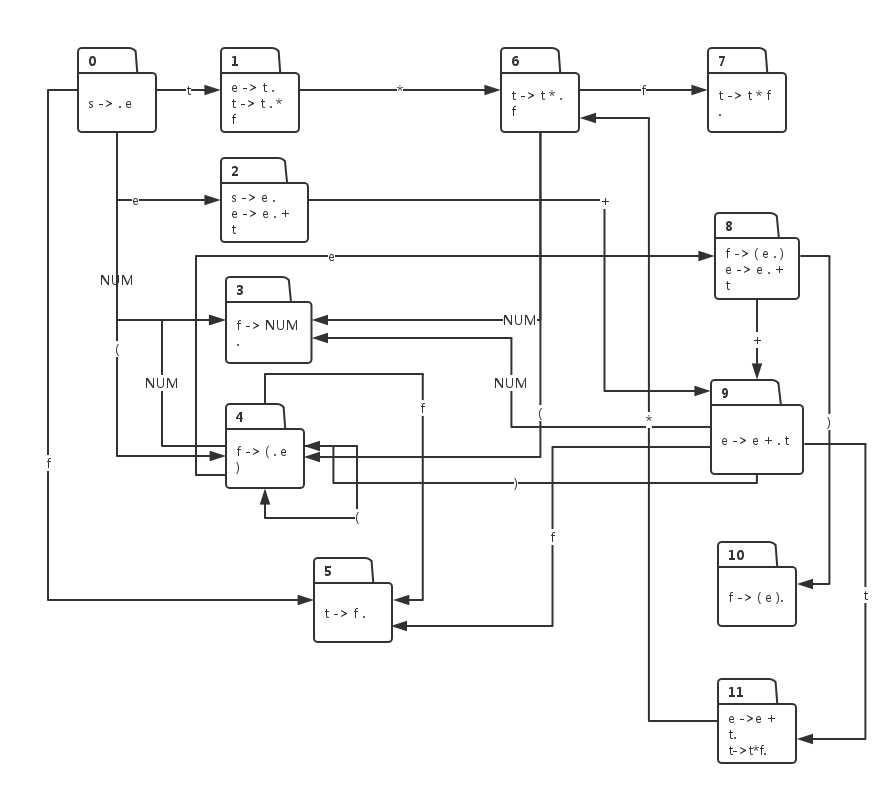上面的图看起来复杂凌乱，只要大家理解了本节描述的算法，上面的状态机图完全可以由程序自动生成。

接下来我们看看，构建算法的具体步骤：
（1） 初始化：状态0是状态机的初始状态，它包含着语法表达式中的起始表达式，也就是编号为0的表达式：
0
s -> . e
表达式我们做了一些更改，就是在表达式 -> 右边, 它跟着一个符号 “.”, 这个点的作用很重要。

（2） 对 . 右边的符号做闭包操作：如果 . 右边的符号是一个非终结符，那么肯定有某个表达式，-> 左边是该非终结符，把这些表达式添加进来，当前，. 右边的非终结符是 e, 于是把 e在 -> 左边的表达式添加进来：
0
s -> . e
e -> . e + t
e -> .t

对于新加进来的表达式，如果 . 右边的非终结符对应的表达式没有加进来，则继续将他们对应的表达式添加进来，根据上面的例子，我们需要把 t 对应的表达式添加进来：
0
s -> . e
e -> . e + t
e -> . t
t ->. t  *  f
t -> . f
继续重复闭包操作，新引入的表达式中，. 右边的非终结符f 对应的表达式还没有引入，于是下一步是引入f 对应的表达式：
0
s -> . e
e -> . e + t
e -> . t
t ->.  t  *  f
t -> .  f
f -> .  (  e  )
f -> .  NUM
由于引入的表达式, . 右边都是终结符，所以闭包操作结束。

（3） 对引入的表达式进行分区：把 . 右边拥有相同非终结符的表达式划入一个分区：
0
e -> . t
t -> . t * f
s -> . e
e -> . e + t
f -> . NUM
f -> . ( e )
t -> . f

把每个分区中的表达式中的 . 右移动一位，形成新的状态节点，例如第一个分区中，表达式中的 . 右移一位后生成新节点1：
1
e -> t .
t ->t . * f

第二个分区表达式， . 右移一位后形成新的节点2：
2
s -> e .
e ->e . + t

第三个分区， . 右移一位后形成新的节点3:
3
f -> NUM .

第四个分区, . 右移动一位后形成新的节点4：
4
f -> ( . e )

第5个分区，. 右移动一位后形成新的节点5
5
t -> f .

（4） 构建原有节点与新生节点间的跳转关系
由于节点1表达式中， . 左边的符号是 t,  所以当状态机处于状态0时，输入时 t 时， 跳转到状态1：
0 – t -> 1

由于节点2表达式中，. 左边的符号是e, 所以当状态机处于状态 0 ，且输入时符号e时，跳转到状态2：
0 – e -> 2

由于节点 3 表达式中， . 左边的符号是 NUM, 所以当状态机处于状态 0 , 输入是符号 NUM时，跳转到状态 3：
0 – NUM -> 3

由于节点4表达式中，. 左边的符号是 (, 所以当状态机处于状态0，输入是 ( 时， 状态机跳转到状态4：
0 – ( -> 4.

由于节点5表达式中，. 左边的符号是f, 所以当状态机处于状态0，输入是符号 f 时，状态机由状态0跳转到状态5：
0 – f -> 5.

对每个新生成的状态节点重复上面的算法，最终将构成给定的状态机图。
展开全文java 编译器
• 该算法利用LR(1)基本集代替LR(1)项集，对于归约状态直接标注归约转移后的状态编号。该分析不含GOTO，基于它的LR(1)语法分析过程一般不需要后入先出栈的辅助。文中的一个实例说明了该分析的有效性。
• LR(0) 步骤 1. 写成 拓广文法 （S’→ S） 2. 活前缀的 DFA（靠近.后面的非终结符） 看状态Ii里面，是不是只存在一个规约项目。如果有两个规约项目，就是规约...例题1（项目集规范族、LR(0)分析） 例题2 ...
LR(0)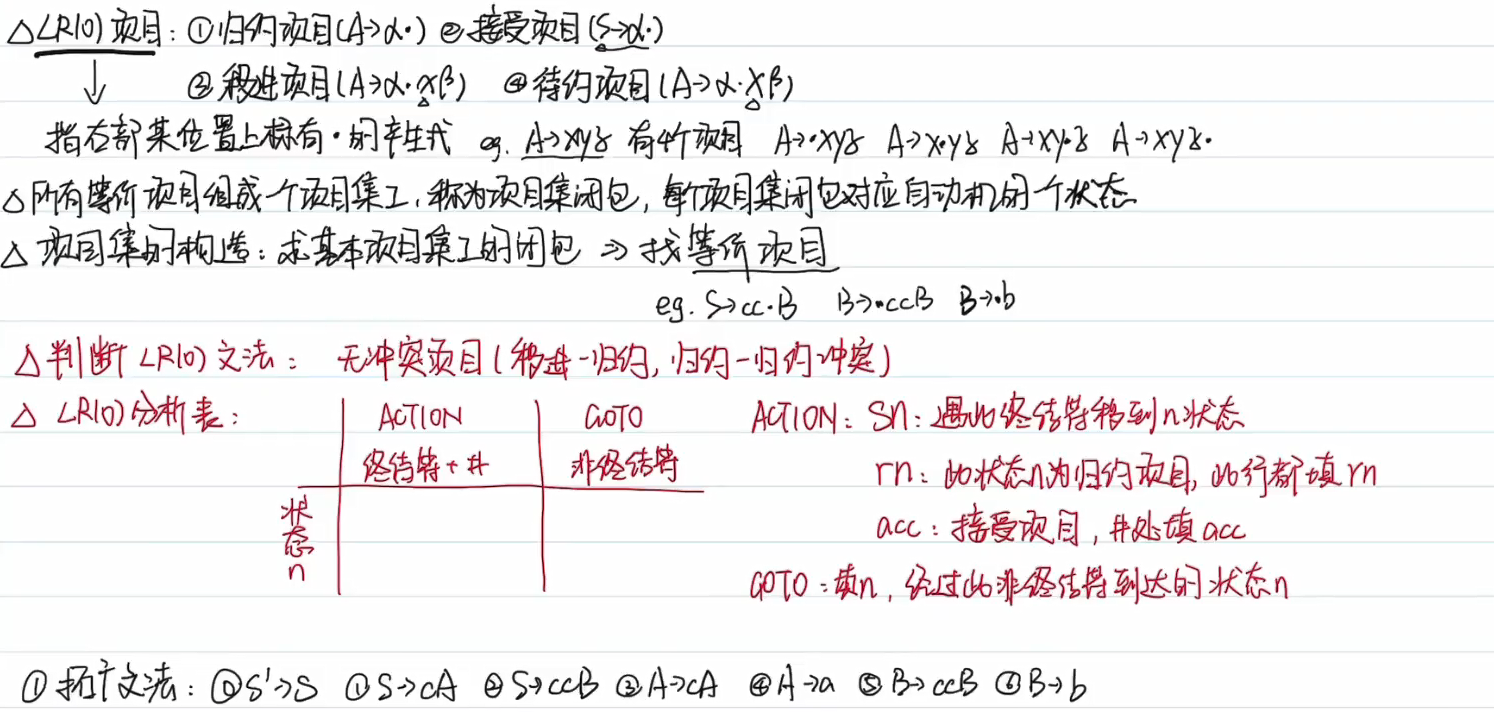步骤
1. 写成 拓广文法 （S’→ S）
2. 活前缀的 DFA（靠近.后面的非终结符）
看状态Ii里面，是不是只存在一个规约项目。如果有两个规约项目，就是规约——规约冲突；如果同时有规约项目和移进项目，就是移进——规约冲突。
3. ACTION表写终结符 + “#”，GOTO表写非终结符
4. GOTO表只填写数字，ACTION表看项目类型
例题1（项目集规范族、LR(0)分析表）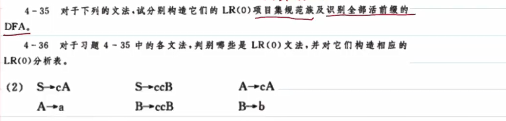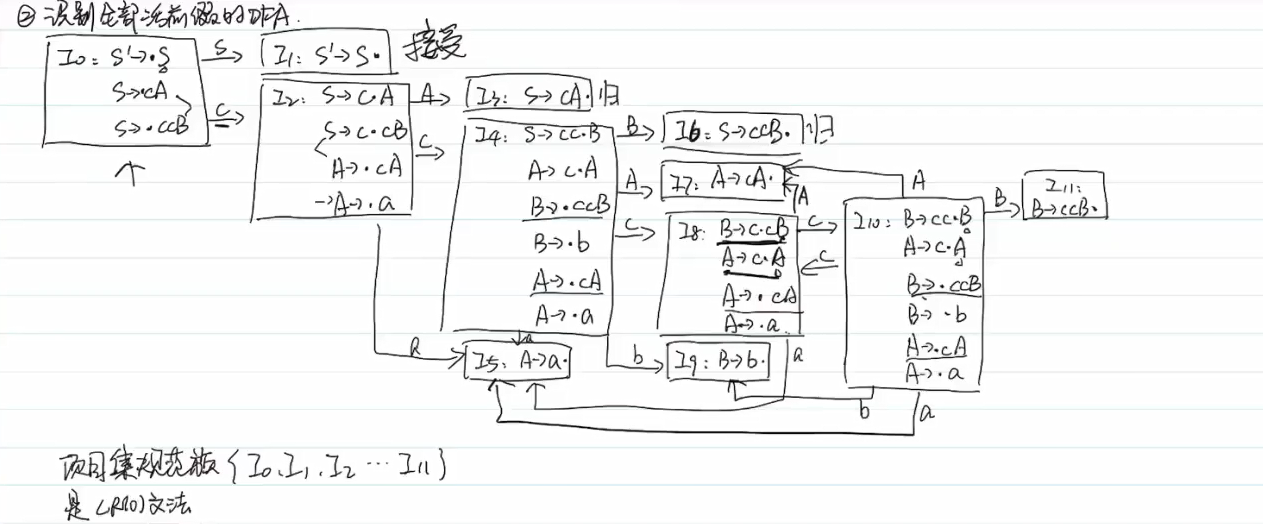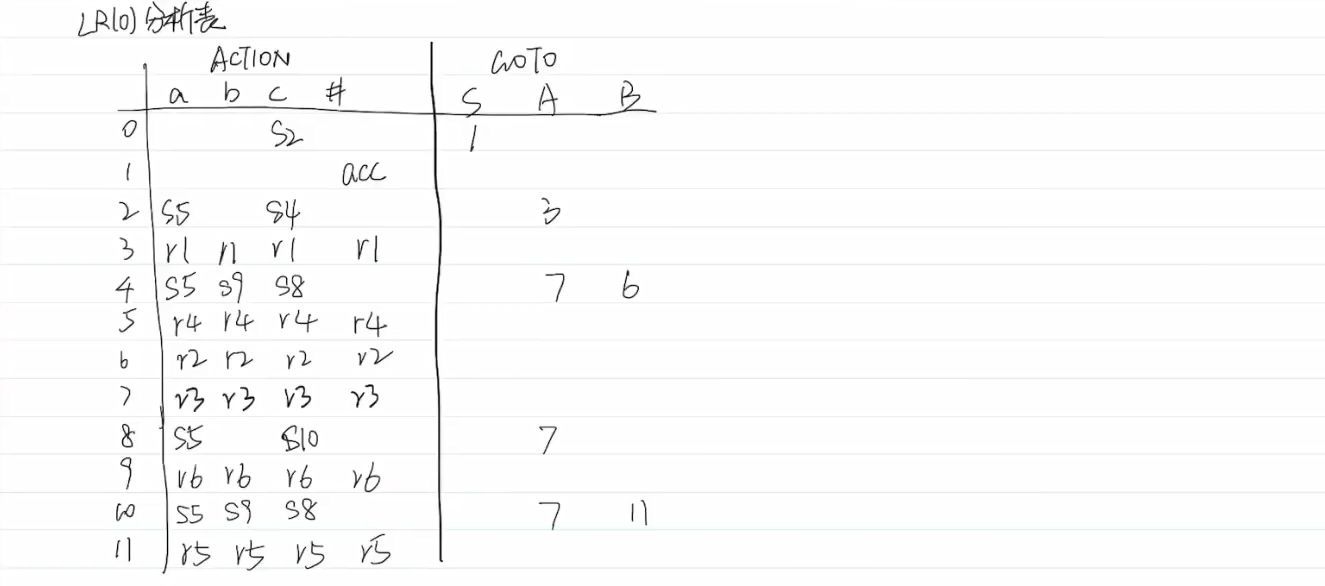例题2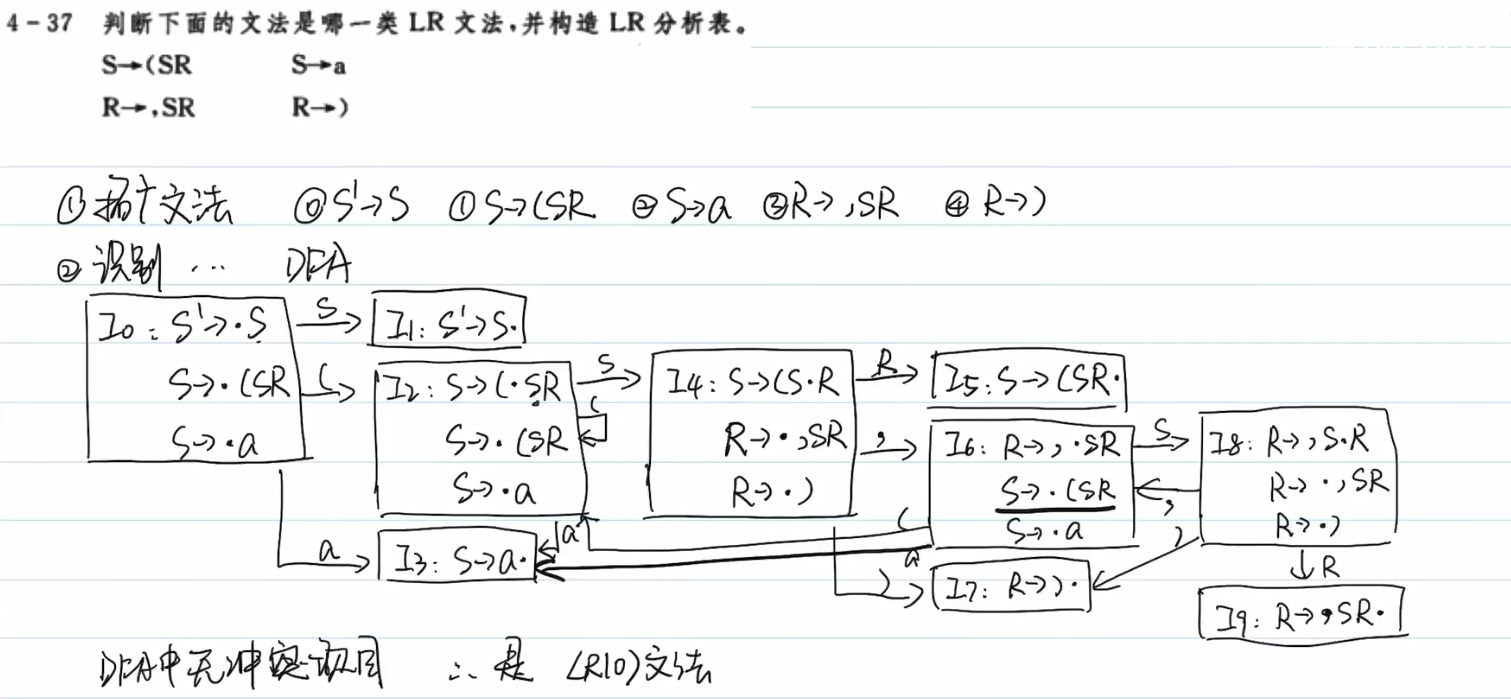展开全文• 《编译原理》LR 分析法与构造 LR(1) 分析的步骤 - 例题解析 笔记 直接做题是有一些特定步骤，有技巧。但也必须先了解一些基本概念，本篇会通过例题形式解释概念，会容易理解和记忆，以及解决类似问题。 如果只想做...
• ## Python实现LR分析

千次阅读 2018-08-29 10:44:33
输入：输入序列w和文法G的LR分析action和goto 输出：若w属于L(G),得到w的规范规约，否则指出一个错误 方法：初始格局为(#0, w#, 驱动器的第一个动作)，其中0是初始状态。令ip指向w#中的第一个终结符，top指向...
• 目录LR(0)LR(0)项目LR(0)的分析基础——增广文法文法中的项目构造LR(0)自动机LR(0)分析构造LR(0)分析过程中的冲突 LR(0) LR(0)项目 LR(0)的分析基础——增广文法 进行LR(0)分析的文法必须先转变成增广文法的形式 ...
• ## LR(0)

2020-05-10 22:51:16
LR(0) : 假如一个文法G的拓广文法G’的活前缀识别自动机的每个状态（项目集）不存在下述情况： (1):既含移进项目又含归约项目 (2) 含多个归约项目 则称G是一个LR(0)文法。换言之，LR(0)文法规范族的每个项目集不包含...
• ## LR分析法的实现

热门讨论 2012-12-22 23:59:34
2、对应的LR分析如下： 状态 ACTION GOTO + * ( ) i # E 0 S2 S3 1 1 S4 S5 acc 2 S2 S3 6 3 r4 r4 r4 r4 4 S2 S3 7 5 S2 S3 8 6 S4 S5 S9 7 r1 S5 r1 r1 8 r2 r2 r2 r2 9 r3 r3 r3 r3 3、编程运用上述LR...
• 算法: 初始状态s放入分析栈顶,输入...规约过程中,产生的非终结符A,与此时栈顶做key,查找goto,将A和goto状态,压入分析栈; LR0是状态中包涵移近还是规约的信息 LR1是根据当前状态和输入确定移近或是规约...